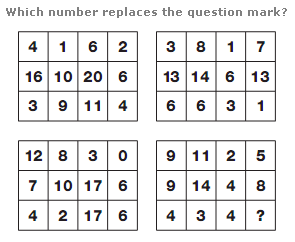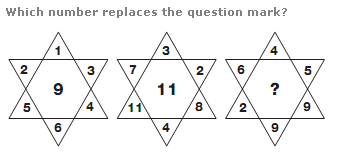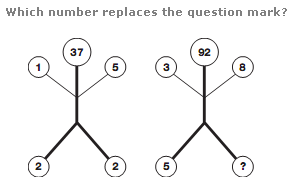# Puzzles - Number puzzles

### Exercise :: Number puzzlesAnswer : 8 Explanation : In each grid, working in columns, add the top and bottom numbers together, and put this sum in the centre square in the grid underneath the original (for the top grids), or the grid above (for the lower grids).Answer : 5 Explanation : In each star, the value at the centre equals the difference between the sum of the top 3 numbers and the sum of the bottom 3 numbers.Answer : 4 Explanation : In each diagram, read the upper and lower pairs of numbers as 2 digit values and add these together to give the result written at the top.